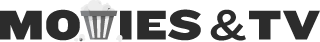## Top users by calculated rep

0

(click on this box to dismiss)

Calculate a basic rep using rep rules. Can be useful for Meta sitesQ&A about the site for movie and tv enthusiasts

``````-- Top  users by calculated rep
-- Calculate a basic rep using rep rules.  Can be useful for Meta sites

WITH VotePoints AS (
SELECT
Posts.OwnerUserId AS UserId,
VoteTypeId,
(CASE
WHEN VoteTypeId = 1 THEN 15                    -- Accepted answer
WHEN VoteTypeId = 2 AND PostTypeId = 1 THEN 10  -- Upvoted question
WHEN VoteTypeId = 2 AND PostTypeId = 2 THEN 10 -- Upvoted answer
WHEN VoteTypeId = 3 THEN -2                    -- Downvote
WHEN VoteTypeId = 9 THEN BountyAmount          -- Earned Bounty
END) AS Points,
(CASE
THEN 1 ELSE 0
END) AS IsCommunityOwned,
(CASE
WHEN (SELECT TOP 1 CreationDate FROM PostHistory WHERE PostHistoryTypeId=17 AND PostHistory.PostId = Votes.PostId ORDER BY CreationDate DESC) > Votes.CreationDate
THEN 1 ELSE 0
END) AS IsBeforeMigration
FROM
INNER JOIN
)

, CalculatedReputation AS (
SELECT
Users.Id AS UserId,
SUM(Points) AS Total,
SUM(CASE
WHEN (VoteTypeId = 2 OR VoteTypeId = 3 OR VoteTypeId = 3)
AND IsCommunityOwned = 1 AND IsBeforeMigration = 0
THEN Points ELSE 0 END) AS LostWiki,
SUM(CASE
WHEN IsBeforeMigration = 1
THEN Points ELSE 0 END) AS LostMigration
FROM
VotePoints
INNER JOIN
Users ON Users.Id = UserId
GROUP BY
Users.Id,
Users.Reputation
)

SELECT TOP 1000
Users.Reputation as [Site Rep],
Total as [Calculated Rep]
FROM
CalculatedReputationHold tight while we fetch your results